# RBSE Maths Class 10 Chapter 1: Vedic Maths Important Questions and Solutions

Vedic Maths Class 10 RBSE Important questions and solutions are given here. All these questions have detailed solutions so that students can easily understand and remember for a long time. The RBSE important questions and solutions of Class 10, provided at BYJU’S, will help the students to cover all the important concepts of mathematics in an effective way.

Vedic Maths Chapter 1 of RBSE Class 10 contains several mathematical operations to be performed using various methods. For example, division, finding the square, cube, square root and cube root. Besides, there are some algebraic formulas that exist to solve the equations using simple formulas.

### RBSE Maths Chapter 1: Exercise 1.1 Textbook Important Questions and Solutions

Question 1: Divide by the Nikhilam method: 1245 ÷ 97

Solution:

Steps for division:

(i) Nearest base of 97 is 100.

(ii) Difference is 97 – 100 = -03

(iii) Write 0 and 3 below 2 and 4 respectively.

(iv) 2 – 0 = 2, Again write the product 06 (i.e. 0 3 and 2) below 4 and 5

(v) By adding, quotient = 12 and remainder = 81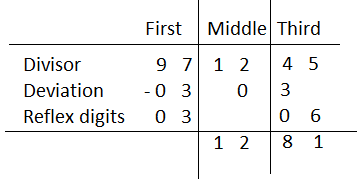Question 2: Divide by the Nikhilam method: 311 ÷ 8

Solution:

Steps for division:

(i) Nearest base of 8 is 10.

(ii) Deviation” 10 – 8 = 2

(iii) Write 2 below 8 and write 3 in the middle column as it is.

(iv) Write product of 2 and 3, i.e. 6 below 1

(v) Add the middle column numbers

(vi) Write product of 2 and 7 below 1 in the third column.

(vii) Add 14 + 1 = 15, 15 > divisor 8

(viii) Adjust the last row with 1 and 8 (product of 1 and 8 is equal to 8).

(ix) Consider the negative sign for 8 to be written below 15.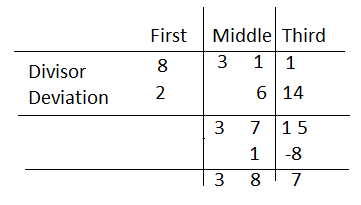Therefore, quotient = 38 and remainder = 7

Question 3: Divide by the Nikhilam method: 1013 ÷ 88

Solution:

Steps for division:

(i) Nearest base of 88 is 100

(ii) Deviatio: 100 – 88 = 12

(iii) Write 1 and 2 below 0 and 1 respectively.

(iv) Add the numbers in the middle column

(v) Multiply deviation 12 with 1 (unit digit in the middle column) and write in the third column

(vi) Add the numbers in the third column.

(vii) Hence, quotient = 11 and remainder = 45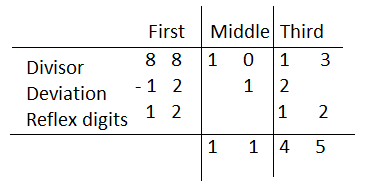Question 4: Find the square of the number 95.

Solution:

Square of 95 by Dwandwa yoga method:

= (9)2 / 2 × 9 × 5 / (5)2

= 81 / 90 / 25

= 81/ 90 / 2 5

= 90/ 2 / 5

= 9025

Question 5: Find the square of the number 204.

Solution:

Square of 204 by Dwandwa yoga method:

= (2)2 / 2 × 2 × 0 / 2 × 2 × 4 + (0)2 / 2 × 0 × 4 / (4)2

= 4 / 0 / 16 / 0 / 16

= 4 / 0 / 16 / 0 / 16

= 4 / 1 / 6 / 1 / 6

= 41616

Question 6: Find the square of the number 225.

Solution:

Square of 225 by Dwandwa yoga method:

= (2)2 / 2 × 2 × 2 / 2 × 2 × 5 + (2)2 / 2 × 2 × 5 / (5)2

= 4 / 8 / 24 / 20 / 25

= 4 / 8 / 24 / 20 / 25

= 4 / 10 / 6 / 2 / 5

= 4/ 10 / 6 / 2 / 5

= 50625

Question 7: Find the cube of the number 51.

Solution:

Cube of 51:

= (5)3 / 3 × 1 × (5)2 / 3 × 5 × (1)2 / (1)3

= 125 / 3 × 25 / 15 / 1

= 125 / 75 / 15 / 1

= 125 / 7 5 / 1 5 / 1

= 132 / 6 / 5 / 1

= 132651

Question 8: Find the cube of the number 63.

Solution:

Cube of 63:

= (6)3 / 3 × 3 × (6)2 / 3 × 6 × (3)2 / (3)3.

= 216 / 9 × 36/ 18 × 9 / 27

= 216 / 324 / 162 / 27

= 216 / 32 4 / 16 2 / 2 7

= 248 / 20 / 4 / 7

= 248 / 2 0 / 4 / 7

= 250 / 0 / 4 / 7

= 250047

Question 9: Find the cube of the number 106.

Solution:

Cube of 106:

= 106 + 2 × (06) / 3 × (06)2 / (06)3

= 106 + 12 / 3 × 36 / 216

= 118 / 108 / 216

= 118 / 1 08 / 2 16

= 119 / 10 / 16

= 1191016

Question 10: Find the cube of the number 18.

Solution:

Cube of 18:

= 18 + 2 × 8 / 3 × (8)2 / (8)3

= 18 + 16 / 3 × 64 / 512

= 34 / 192 / 512

= 34 / 19 2 / 51 2

= 53 / 53 / 2

= 53 / 5 3 / 2

= 58 / 3 / 2

= 5832

Question 11: Divide by Dhvajanka method: 4532 ÷ 112

Solution:

Steps for division:

(i) Write the divisor and dividend as shown below.

(ii) When 45 is divided by 11, quotient is 4 and remainder is 1.

(iii) Write 4 as the result of the first number in the middle column and write 1 between 5 and 3 in the next row.

(iv) Now, 13 – (2 × 4) = 13 – 8 = 5 and 5 is divisible by 11, quotient is 0 and remainder is 5.

(v) Write 5 on the left below 2 in the third column and 0 in the result row of the middle column.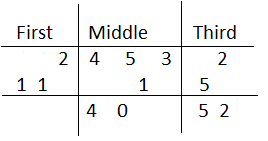Therefore, quotient = 40 and remainder = 52

Question 12: Divide by Dhvajanka method: 98765 ÷ 87

Solution:

Steps for division:

(i) Write the digits of both dividend and divisor as shown below.

(ii) 9 ÷ 8, quotient is 1, remainder is 1 and 18 – (7 × 1) = 18 – 7 = 11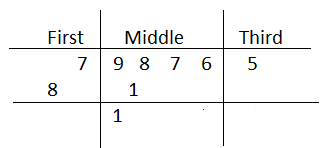(iii) 11 ÷ 8, quotient is 1, remainder is 3 and 37 – (7 × 1) = 37 – 7 = 30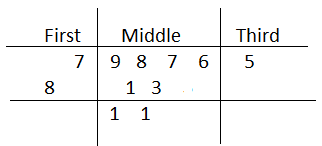(iv) 30 ÷ 8, quotient is 3, remainder is 6 and 66 – (7 × 3) = 66 – 21 = 45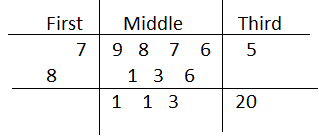(v) 45 ÷ 8, quotient is 5, remainder is 5 and 55 – (7 × 5) = 55 – 35 = 20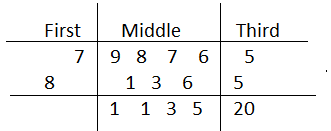Therefore, quotient = 1135 and remainder = 20

Question 13: Divide by Dhvajanka method: 2101532 ÷ 879

Solution:

Steps for division:

(i) Write the digits of both dividend and divisor as shown below.

(ii) Follow the below steps for the calculation of quotients and remainders in each case.

21 ÷ 8, quotient is 2, remainder is 5 and 50 – (7 × 2) = 50 – 14 = 36

36 ÷ 8, quotient is 4, remainder is 4 and 41 – (7 × 4 + 2 × 9) = 41 – 46 = -5 (ignore)

36 ÷ 8, quotient is 3, remainder is 12 and 121 – (7 × 3 + 2 × 9) = 121 – 39 = 82

82 ÷ 8, quotient is 9, remainder is 10 and 105 – (7 × 9 + 7 × 3) = 105 – 90 = 15

15 ÷ 8, quotient is 1, remainder is 7 and 73 – (7 × 1 + 9 × 9) = 73 – 88 = -15 (ignore)

15 ÷ 8, quotient is 0, remainder is 15 and 153 – (7 × 0 + 9 × 9) = 153 – 81 = 72

722 – (9 × 0) = 722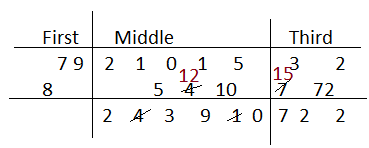Therefore, quotient = 2390 and remainder = 722

Question 14: Divide by Paravartya Yojayet method: 1154 ÷ 103

Solution:

Steps for division:

(i) Take the nearest base of 103, i.e. 100

(ii) Difference: 103 – 100 = 03

(iii) Take the bar for 3 and write as shown below.

(iv) Write 03 in the second row and product of 1 and 03 in the third column & third row.

(v) Subtract the digits to get the final result.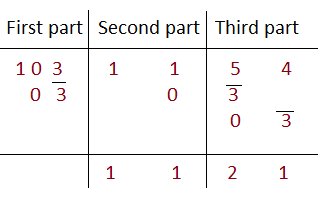Therefore, quotient = 11 and remainder = 21

Question 15: Divide by Paravartya Yojayet method: 1358 ÷ 113

Solution:

Steps for division:

(i) Take the nearest base of 113, i.e. 100

(ii) Difference: 113 – 100 = 13

(iii) Take the bar for 1 and 3 then write as shown below.

(iv) Write 13 in the second row and subtract the digits in the middle column.

(v) Write the product of 2 and 13, i.e. 26 in the third column & third row.

(iv) Subtract the digits to get the final result.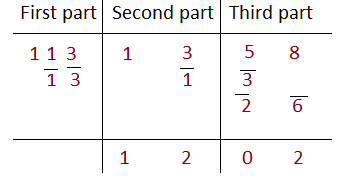Question 16: Divide by Paravartya Yojayet method: 1432 ÷ 88

Solution:

Steps for division:

(i) Take the nearest base of 88, i.e. 100

(ii) Difference: 100 – 88 = 12

(iii) Write 1 2 in the second row and add the digits in the middle column.

(iv) Write the product of 5 and 13 (consider individual digits), i.e. 5 and 10 in the third column & third row.

(v) Add the digits to get the result such as 15 and 112.

(vi) 112 > 88, so place 1 below 5 and -88 in the last column.

(vii) Add the digits in the middle column and subtract the third column.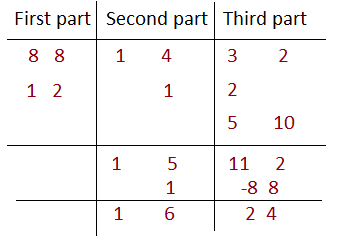Therefore, quotient = 16 and remainder = 24

### RBSE Maths Chapter 1: Additional Important Questions and Solutions

Question 1: Find the square of 62 by Dwandwa yoga method.

Solution:

Square of 62 by Dwandwa yoga method:

= (6)2 / 2 × 6 × 2 / (2)2

= 36 / 24 / 4

= 36/ 24 / 4

= 38 / 4 / 4

= 3844

Question 2: Solve: (x + 1)(x + 2) = (x – 5)(x – 6)

Solution:

Given,

(x + 1)(x + 2) = (x – 5)(x – 6)

We know that the solution of (x + a)(x + b) = (x + c)(x + d) is given by an algebraic formula, x = (cd – ab)/(a + b – c – d)

x = [(-5) × (-6) – 1 × 2] / [(1 + 2 -(-5) – (-6)]

= (30 – 2)/ (1 + 2 + 5 + 6)

= 28/14

= 2

Therefore, the value of x is 2.

Question 3: Find the cube of 42 by Upsutra Anurupyena.

Solution:

Cube of 42 by Upsutra Anurupyena method:

I II III IV

43 42 × 2 4 × 22 23

64 32 16 8

+64 +32

64 96 48 8

= 64 / 96 / 48 / 8

= 64 / 9 6 / 4 8 / 8

= 73 / 10 / 8 / 8

= 73 / 1 0 / 8 / 8

= 74 / 0 / 8 / 8

= 74088

Question 4: Find the value of 31 1/6 × 31 5/6 by using Ekadhikena Purvena Sutra.

Solution:

31 1/6 × 31 5/6 = 31 × 32 / (1/6) × (5/6)

= 992 / (5/36)

= 992 5/36

Question 5: Solve: 1/(x -3) + 1/(x – 7) = 1/(x – 1) + 1/(x – 9)

Solution:

Sum of the denominators in LHS = x – 3 + x – 7 = 2x – 10

Sum of the denominators in RHS = x – 1 + x – 9 = 2x – 10

So, 2x – 10 = 0

2x = 10

x = 10/2 = 5

Question 6: Find the square root of 6889 using Dwandwa Yoga Method.

Solution:

Square root using Dwandwa Yoga Method: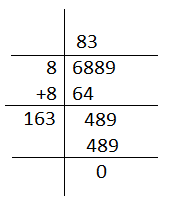Quotient = 83

Therefore, the square root of 6889 is 83.

Question 7: Solve by vedic method: (3x + 2) / (3x + 7) = (3x + 7) / (3x + 2)

Solution:

Given,

(3x + 2) / (3x + 7) = (3x + 7) / (3x + 2)

Sum of the numerators = 3x + 2 + 3x + 7 = 6x + 9

Sum of the denominators = 3x + 7 + 3x + 2 = 6x + 9

Sum of numerators and denominators is the same.

So, 6x + 9 = 0

6x = -9

x = -9/6 = -3/2

Question 8: Solve: 6/(x + 2) + 5/(x + 3) = 0

Solution:

Given,

6/(x + 2) + 5/(x + 3) = 0

We know that the solution of the equation of the form m/(x + a) + n/(x + b) = 0 is x = -(mb + na)/(m + n)

Thus,

x = -(6 × 3 + 5 × 2)/(6 + 5)

= -(18 + 10)/11

= -28/11

Question 9: Find the cube root of 314432 by vedic method.

Solution: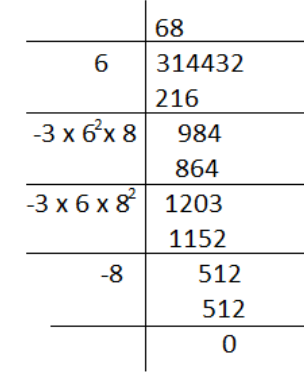Hence, quotient = 68

Therefore, the cube root of 314432 is 68.